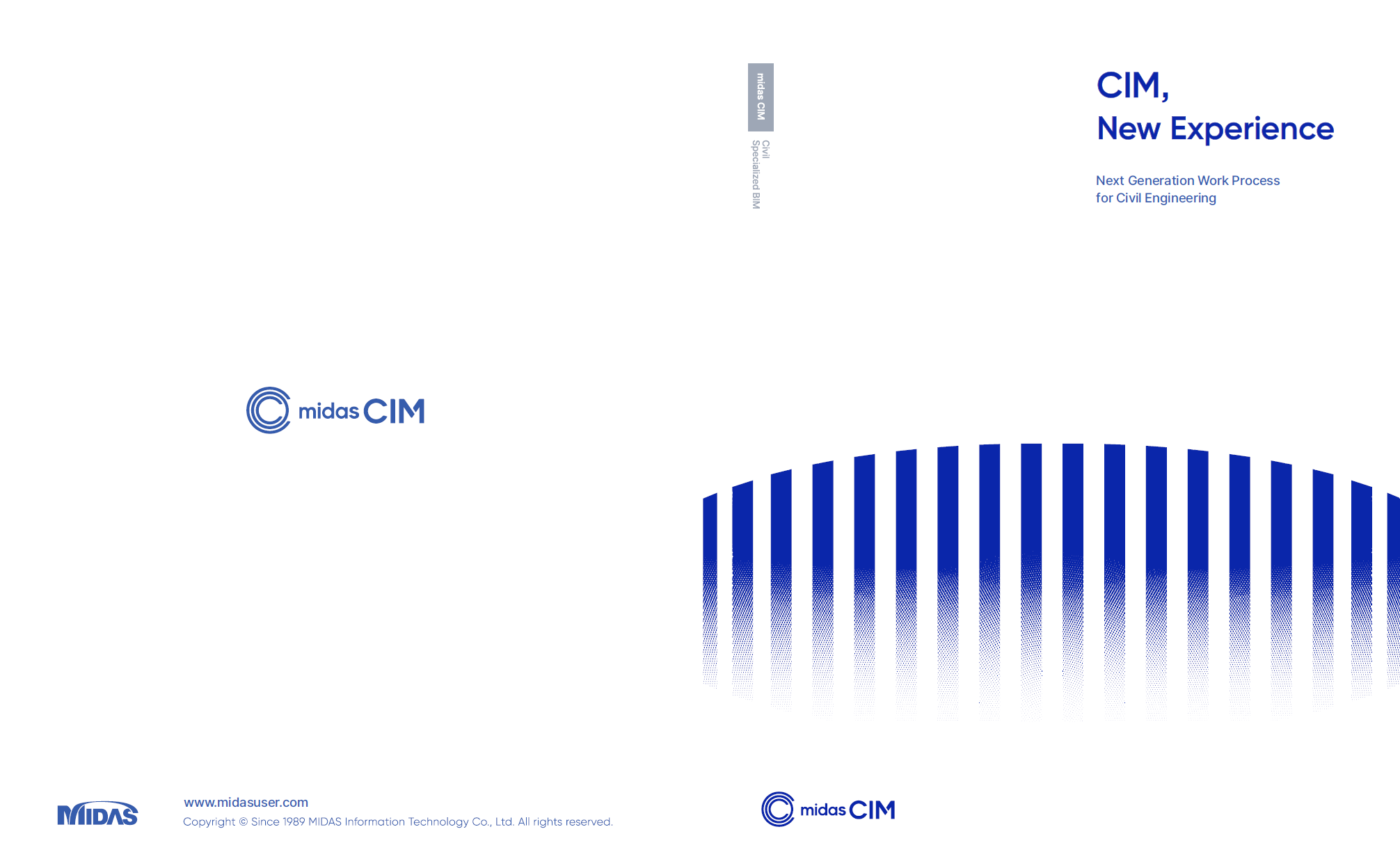BLOG TIPS & TUTORIALS

*Click the content to move to the section

### I. Cassion Modeling Using midas CIM

1. Introduction

In this project application case, we will look at creating a caisson model using midas CIM. This is an area of great interest to many engineers in the field of port and harbor infrastructure.

We will introduce how to use CIM to construct a caisson BIM model with a complex 3D shape, which has various compartments, haunches, and shear key, at LOD 300 level.

2. Description

Many engineers may be more familiar with 2D cross-sections than 3D models.

Therefore, we will explore how to create a 3D CIM model by applying 2D cross-sections.

First, we distinguish the parts of the caisson that have continuity of cross-sections.

Typically, the lower plate and toe of the caisson have horizontal continuity, while the wall, slit part, and shear key have vertical continuity, as shown in the figure below.

Therefore, the modeling needs to be performed by considering the continuity of each cross-section.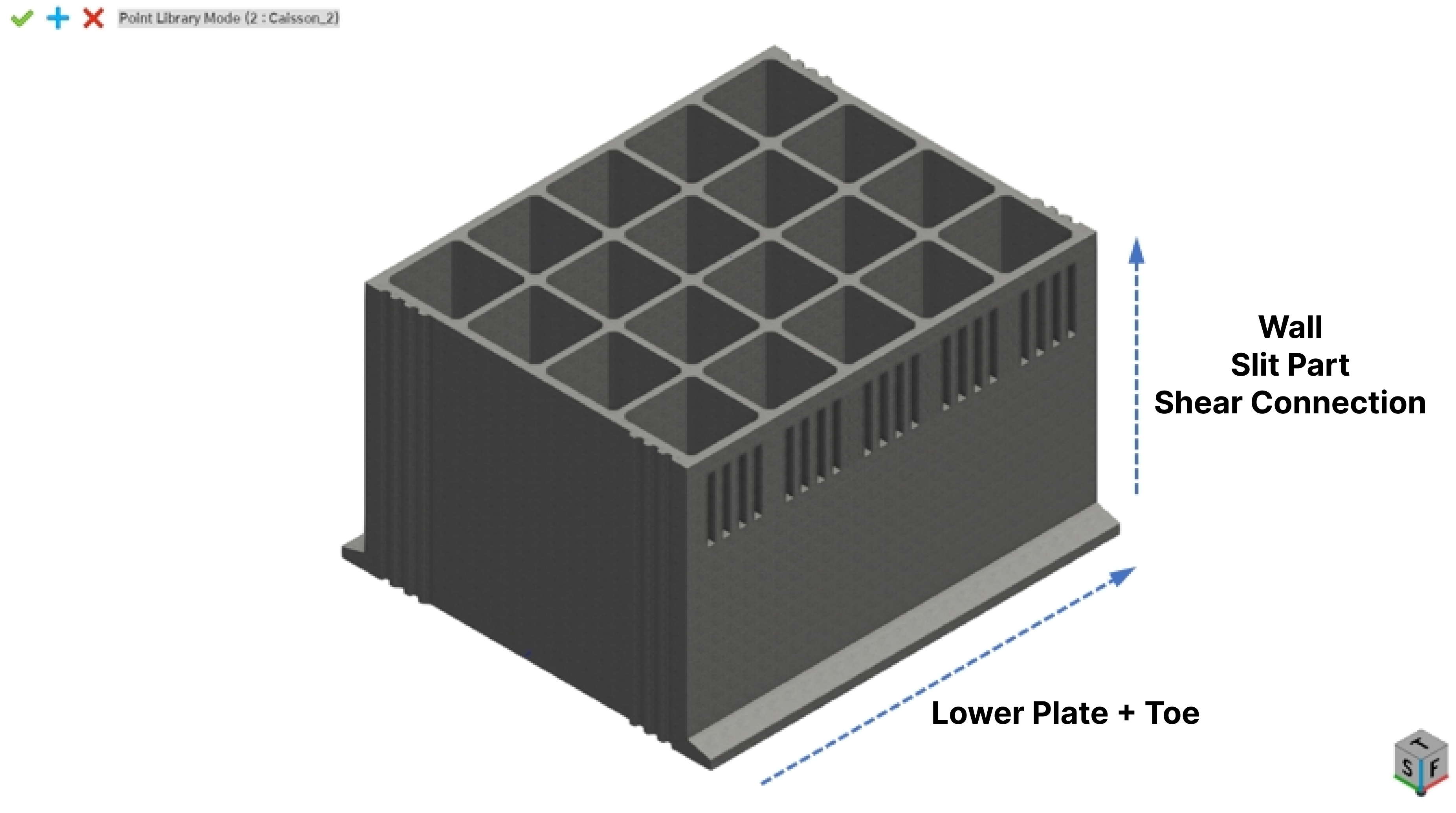The order of modeling using midas CIM for the caisson is as follows:

1) Create a 2D cross-section using midas Drafter or create a CIM User Section directly
2) Section Export using the CIMUSERSECTION command in midas Drafter
3) Import 2D sections from CIM in the CIM Point Library Mode
4) Create a General Member: apply Tapered Section for tapered cross-sections
5) Assign Single Points Linked Entity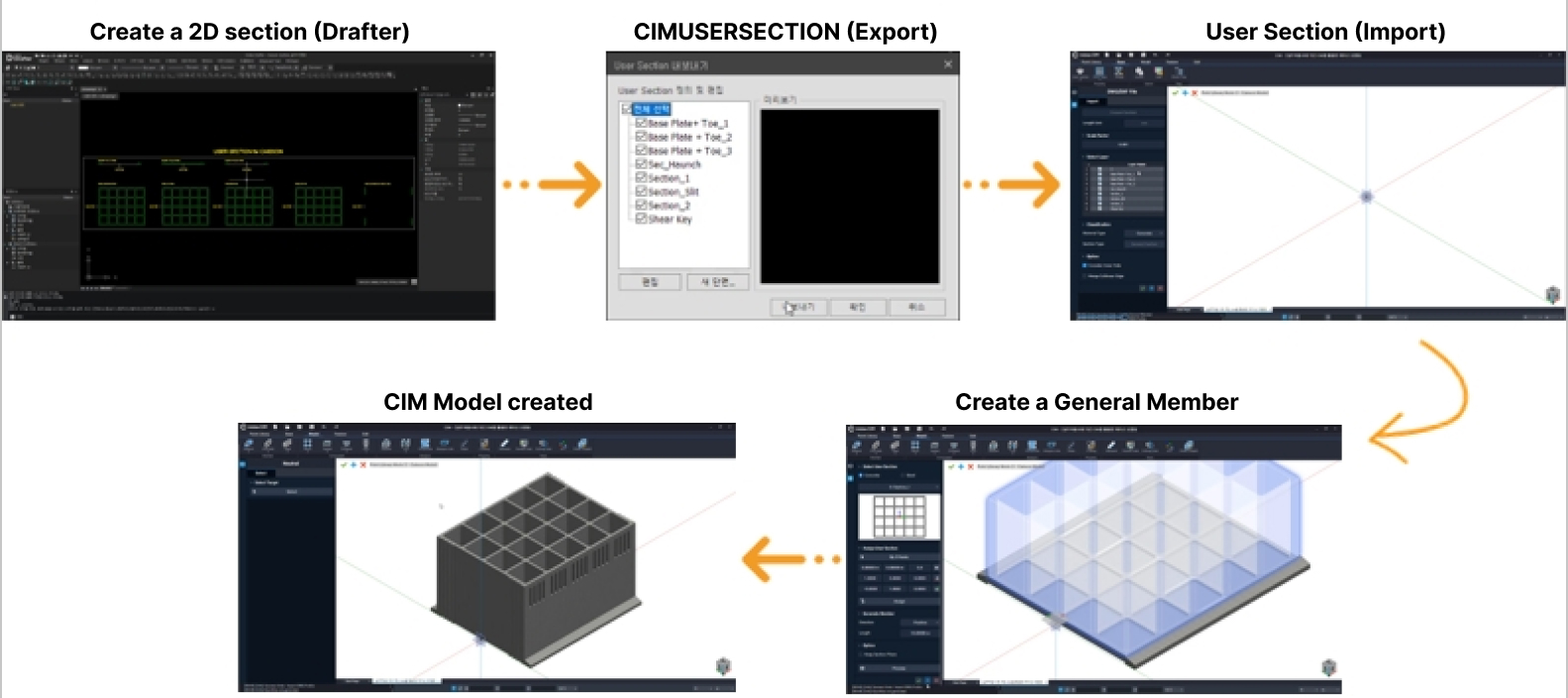The following video shows an example of modeling a caisson using CIM according to the above process:

[Caisson Modeling Using midas CIM]

The height and length of the slit part of the caisson may change depending on the range of waves caused by the tide level and installation depth.

In this case, midas CIM can easily cope with the height variation of the caisson model by applying Multi-Points Linked Entity.

You can change the overall height of the caisson by changing the coordinates of the structure, as shown in the video below.

[Changing the Caisson Model Using Multi-Points Linked Entity]

3. Concluding Remarks

How do you feel about creating a caisson model using midas CIM? Do you think creating a 3D model of a caisson is easier than you expected?

The main points of this practical application case are as follows:

1) Select a 2D User Section for each part, considering the continuity of cross-sections

2) Create a 3D CIM model using midas CIM by applying the User Section

3) Cope with the height variation of the caisson model by applying Multi-Points Linked Entity

If you apply the above three items to caisson modeling, you can easily create caisson models of all shapes used in the port industry.

## II. Structural Analysis Model Creation Using midas CIM

1. Introduction

In this part of the article, we will show how to create a model for structural analysis using CIM, and how to link it to midas Civil and GTS NX.

2. Description

2.1 2D Plate Element (with midas/Civil)

For the structural analysis of a caisson, 2D plate elements are usually required to calculate the moment and shear force for determining the amount of reinforcement.

With midas CIM, it is possible to create 2D plate elements easily using 2D plate lines.

In this practical application case, the thickness of each part was assumed as follows for the generation of a 2D Plate Line.

Front wall, rear wall, side wall: 500.0mm

Lower plate: 600. 0mm

The procedure for linking the analysis between midas CIM and midas Civil is as follows:

1) Create a CIM model: refer to <Caisson Modeling Using CIM> above.
2) Create 2D plate lines (using Drafter or CIM): set the thickness of each member
3) Set up the analysis case: define element mesh size, generation method, etc.
4) Link the midas Civil model
5) Apply loads and design conditions (in midas Civil)
6) Verify cross-sectional forces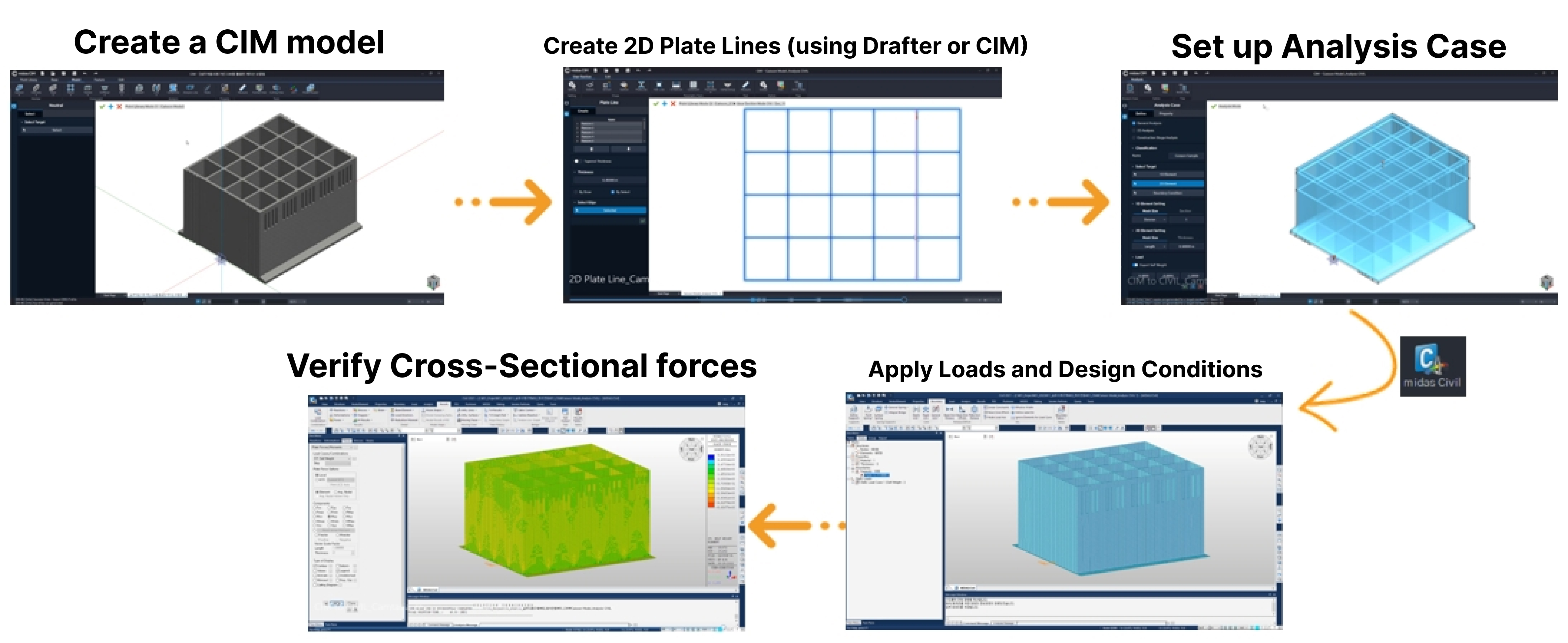The following video shows an example of using CIM to create a 2D plate element caisson model for structural analysis in midas Civil, following the above steps:

[Caisson modeling using midas CIM]

2.2 3D Solid Element (with GTS NX)

Firstly, for smooth element mesh sharing, the models created based on each cross-section are combined into a single model using the Union command, as shown in the following figure.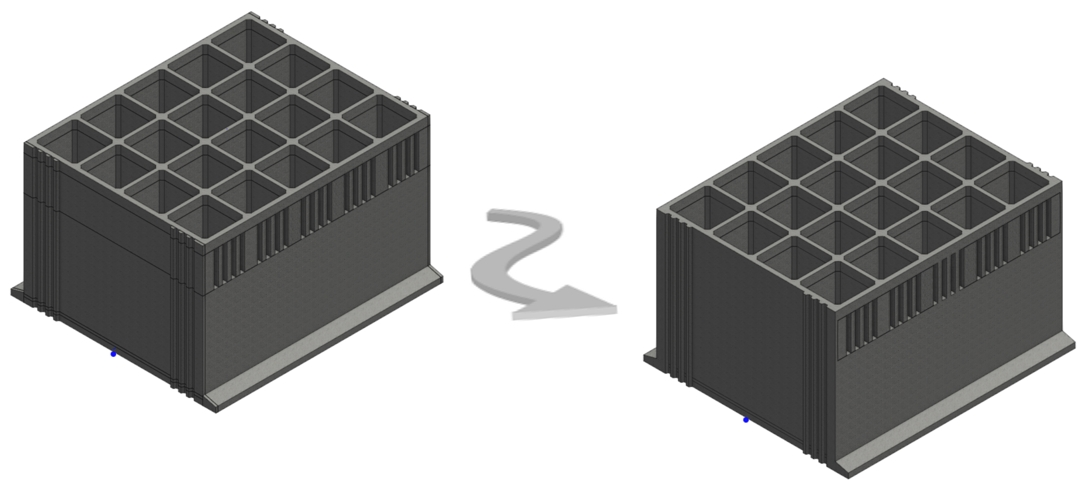The model created using midas CIM can be exported as a Parasolid model and opened directly in GTS NX. Using the geometry model loaded into GTS NX, 3D solid elements can be easily generated, as shown in the following figure.

The generated element mesh shows that the node sharing is well executed.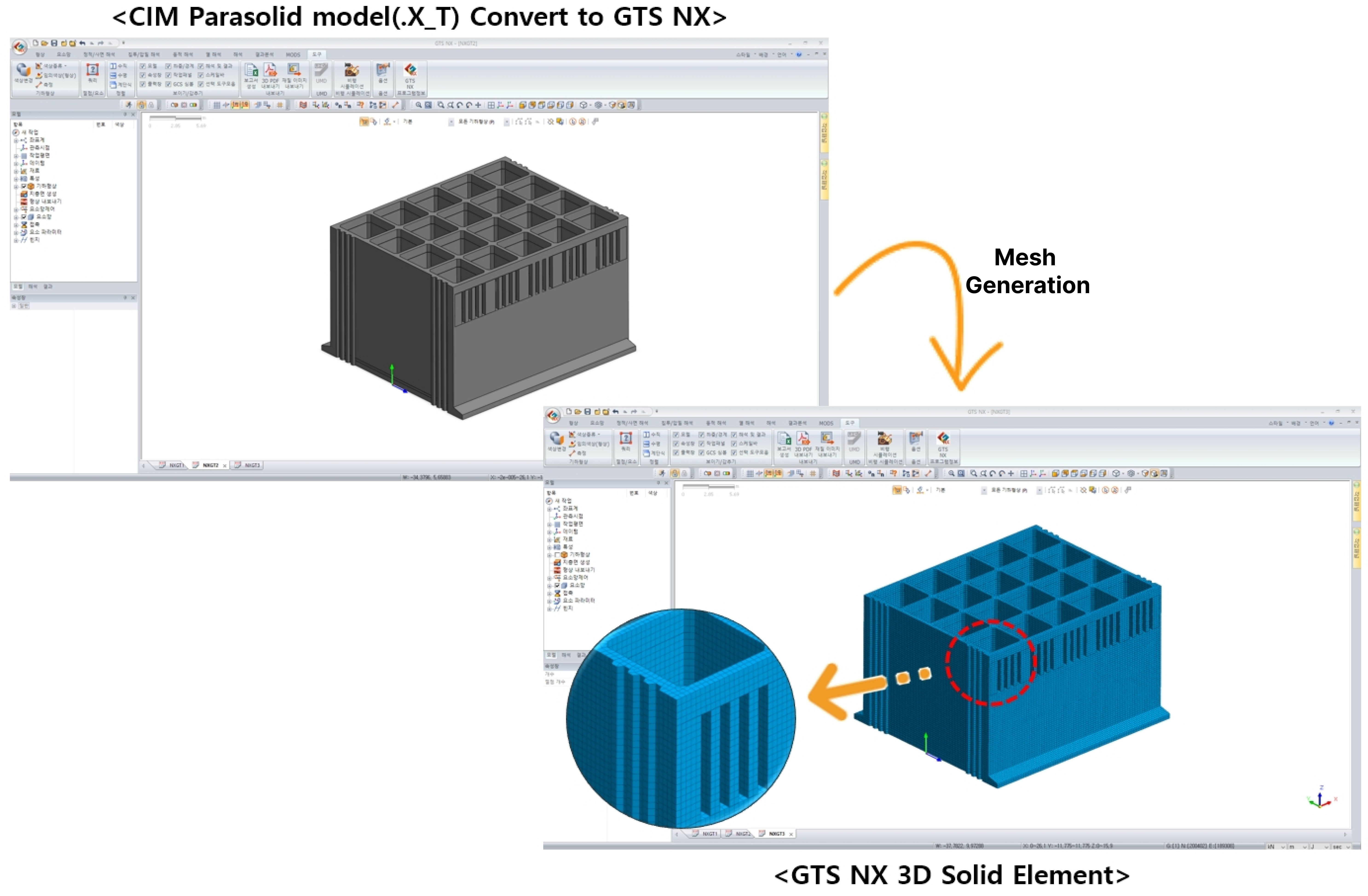3. Concluding Remarks

midas CIM allows direct integration of the generated BIM model into the structural analysis program, midas Civil.

Furthermore, it can easily generate the geometric shape required for creating 3D solid element models for GTS NX analysis.

This practical application example shows that the BIM model can not only display the 3D shape of the structure, but can also be used directly for structural analysis.

We hope that you will now consider using midas CIM for generating complex structural analysis models.

We will continue to introduce practical application examples of CIM for promoting the activation of BIM in civil engineering!